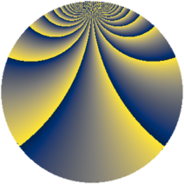# Properties

 Label 1140.2.bdLevel $1140$ Weight $2$ Character orbit 1140.bd Rep. character $\chi_{1140}(11,\cdot)$ Character field $\Q(\zeta_{6})$ Dimension $320$ Sturm bound $480$

# Related objects

## Defining parameters

 Level: $$N$$ $$=$$ $$1140 = 2^{2} \cdot 3 \cdot 5 \cdot 19$$ Weight: $$k$$ $$=$$ $$2$$ Character orbit: $$[\chi]$$ $$=$$ 1140.bd (of order $$6$$ and degree $$2$$) Character conductor: $$\operatorname{cond}(\chi)$$ $$=$$ $$228$$ Character field: $$\Q(\zeta_{6})$$ Sturm bound: $$480$$

## Dimensions

The following table gives the dimensions of various subspaces of $$M_{2}(1140, [\chi])$$.

Total New Old
Modular forms 496 320 176
Cusp forms 464 320 144
Eisenstein series 32 0 32

## Trace form

 $$320q - 2q^{4} - 4q^{9} + O(q^{10})$$ $$320q - 2q^{4} - 4q^{9} - 2q^{10} - 8q^{13} + 2q^{16} - 8q^{24} + 160q^{25} + 16q^{30} + 44q^{33} + 10q^{34} + 28q^{36} - 16q^{37} - 28q^{40} - 2q^{42} - 8q^{46} + 18q^{48} - 368q^{49} + 16q^{52} + 30q^{54} - 24q^{57} + 32q^{58} + 40q^{61} - 32q^{64} - 12q^{66} - 12q^{70} - 16q^{72} - 36q^{76} - 76q^{78} + 20q^{81} + 40q^{82} - 24q^{84} + 16q^{85} - 104q^{88} + 18q^{90} - 36q^{94} + 156q^{96} + 24q^{97} + O(q^{100})$$

## Decomposition of $$S_{2}^{\mathrm{new}}(1140, [\chi])$$ into newform subspaces

The newforms in this space have not yet been added to the LMFDB.

## Decomposition of $$S_{2}^{\mathrm{old}}(1140, [\chi])$$ into lower level spaces

$$S_{2}^{\mathrm{old}}(1140, [\chi]) \cong$$ $$S_{2}^{\mathrm{new}}(228, [\chi])$$$$^{\oplus 2}$$